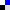Bookmark this on Delicious
 Memento Pattern - Calculator Example - Java Sourcecode

Java Source Code Example for the Memento Pattern - Calculator

This simple example is a calculator that finds the result of addition of two numbers, with the additional option to undo last operation and restore previous result.The code below shows the memento object interface to caretaker. Note that this interface is a placeholder only and has no methods to honor encapsulation in that the memento is opaque to the caretaker.

 ```package memento; /** * Memento interface to CalculatorOperator (Caretaker) */ public interface PreviousCalculationToCareTaker { // no operations permitted for the caretaker } ```

The code below shows the Memento to Originator interface; note that this interface provides the necessary methods for the originator to restore its original state.

 ```package memento; /** * Memento Interface to Originator * * This interface allows the originator to restore its state */ public interface PreviousCalculationToOriginator { public int getFirstNumber(); public int getSecondNumber(); } ```

The code below shows the memento implementation, note that the memento must implement two interfaces, the one to the caretaker as well as the one to the originator.

 ```package memento; /** * Memento Object Implementation * * Note that this object implements both interfaces to Originator and CareTaker */ public class PreviousCalculationImp implements PreviousCalculationToCareTaker, PreviousCalculationToOriginator { private int firstNumber; private int secondNumber; public PreviousCalculationImp(int firstNumber, int secondNumber) { this.firstNumber = firstNumber; this.secondNumber = secondNumber; } @Override public int getFirstNumber() { return firstNumber; } @Override public int getSecondNumber() { return secondNumber; } } ```

The code below shows the calculator interface which is the originator interface

 ```package memento; /** * Originator Interface */ public interface Calculator { // Create Memento public PreviousCalculationToCareTaker backupLastCalculation(); // setMemento public void restorePreviousCalculation(PreviousCalculationToCareTaker memento); // Actual Services Provided by the originator public int getCalculationResult(); public void setFirstNumber(int firstNumber); public void setSecondNumber(int secondNumber); } ```

The code below shows the Calculator implementation which is the originator implementation. Note that the backupLastCalculation method corresponds to createMemento() method discussed previously, in this method the memento object is created and all originator state is saved to the memento. Also note that the method restorePreviousCalculation() method corresponds to setMemento() method . Inside this method the logic to restore the previous state is executed.

 ```package memento; /** * Originator Implementation */ public class CalculatorImp implements Calculator { private int firstNumber; private int secondNumber; @Override public PreviousCalculationToCareTaker backupLastCalculation() { // create a memento object used for restoring two numbers return new PreviousCalculationImp(firstNumber,secondNumber); } @Override public int getCalculationResult() { // result is adding two numbers return firstNumber + secondNumber; } @Override public void restorePreviousCalculation(PreviousCalculationToCareTaker memento) { this.firstNumber = ((PreviousCalculationToOriginator)memento).getFirstNumber(); this.secondNumber = ((PreviousCalculationToOriginator)memento).getSecondNumber(); } @Override public void setFirstNumber(int firstNumber) { this.firstNumber = firstNumber; } @Override public void setSecondNumber(int secondNumber) { this.secondNumber = secondNumber; } } ```

The code below shows the calculator driver which simulates a user using the calculator to add numbers, the user calculates a result, then enters wrong numbers, he is not satisfied with the result and he hits Ctrl + Z to undo last operation and restore previous result.

 ```package memento; /** * CareTaker object */ public class CalculatorDriver { public static void main(String[] args) { // program starts Calculator calculator = new CalculatorImp(); // assume user enters two numbers calculator.setFirstNumber(10); calculator.setSecondNumber(100); // find result System.out.println(calculator.getCalculationResult()); // Store result of this calculation in case of error PreviousCalculationToCareTaker memento = calculator.backupLastCalculation(); // user enters a number calculator.setFirstNumber(17); // user enters a wrong second number and calculates result calculator.setSecondNumber(-290); // calculate result System.out.println(calculator.getCalculationResult()); // user hits CTRL + Z to undo last operation and see last result calculator.restorePreviousCalculation(memento); // result restored System.out.println(calculator.getCalculationResult()); } } ```